# Solidworks Macro - Edit Circular Sketch Pattern

## Introduction

In this post, I tell you about how to Edit Circular Sketch Pattern using Solidworks VBA Macros in a Sketch.

In this post, I explain about `EditCircularSketchStepAndRepeat` method from Solidworks `SketchManager` object.

This method is most updated method, I found in Solidworks API Help.

So use this method if you want to edit existing Circular Sketch Pattern.

This post is a little different of previous Solidworks Macro - Circular Sketch Pattern post.

There are 2 changes I have made, which I am going to use on future posts also.

These change are explain below:

• In this post I used `code sample` from General - Fix Unit Issue post to fix unit conversion issue and show how to use it.

• In input parameter of `EditCircularSketchStepAndRepeat` method, I passed variables not direct values. This helps us to maintain the code and modification of existing code is simple.

## Video of Code on YouTube

Please see below video on how to Edit Circular Sketch Pattern from Solidworks VBA Macros.

Please note that there are no explaination in the video.

Explaination of each line and why we write code this way is given in this post.

## Code Sample

Below is the `code` sample to edit Circular Sketch Pattern.

``````Option Explicit

' Create variable for Solidworks application
Dim swApp As SldWorks.SldWorks

' Create variable for Solidworks document
Dim swDoc As SldWorks.ModelDoc2

' Boolean Variable
Dim BoolStatus As Boolean

' Create variable for Solidworks Sketch Manager
Dim swSketchManager As SldWorks.SketchManager

' Create Variable for Solidworks Sketch Segment
Dim swSketchSegment As SldWorks.SketchSegment

' Main function of our VBA program
Sub main()

' Set Solidworks variable to Solidworks application
Set swApp = Application.SldWorks

' Create string type variable for storing default part location
Dim defaultTemplate As String

' Set value of this string type variable to "Default part template"
defaultTemplate = swApp.GetUserPreferenceStringValue(swUserPreferenceStringValue_e.swDefaultTemplatePart)

' Set Solidworks document to new part document
Set swDoc = swApp.NewDocument(defaultTemplate, 0, 0, 0)

'-----------------------UNIT CONVERSION----------------------------------------

' Local variables used as Conversion Factors
Dim LengthConversionFactor As Double
Dim AngleConversionFactor As Double

' Use a Select Case, to get the length of active Unit and set the different factors
Select Case swDoc.GetUnits(0)       ' GetUnits function gives us, active unit

Case swMETER    ' If length is in Meter
LengthConversionFactor = 1
AngleConversionFactor = 1

Case swMM       ' If length is in MM
LengthConversionFactor = 1 / 1000
AngleConversionFactor = 1 * 0.01745329

Case swCM       ' If length is in CM
LengthConversionFactor = 1 / 100
AngleConversionFactor = 1 * 0.01745329

Case swINCHES   ' If length is in INCHES
LengthConversionFactor = 1 * 0.0254
AngleConversionFactor = 1 * 0.01745329

Case swFEET     ' If length is in FEET
LengthConversionFactor = 1 * (0.0254 * 12)
AngleConversionFactor = 1 * 0.01745329

Case swFEETINCHES     ' If length is in FEET & INCHES
LengthConversionFactor = 1 * 0.0254  ' For length we use sama as Inch
AngleConversionFactor = 1 * 0.01745329

Case swANGSTROM        ' If length is in ANGSTROM
LengthConversionFactor = 1 / 10000000000#
AngleConversionFactor = 1 * 0.01745329

Case swNANOMETER       ' If length is in NANOMETER
LengthConversionFactor = 1 / 1000000000
AngleConversionFactor = 1 * 0.01745329

Case swMICRON       ' If length is in MICRON
LengthConversionFactor = 1 / 1000000
AngleConversionFactor = 1 * 0.01745329
End Select

'----------------------------------------------------------------

' Select Front Plane
BoolStatus = swDoc.Extension.SelectByID2("Front Plane", "PLANE", 0, 0, 0, False, 0, Nothing, swSelectOption_e.swSelectOptionDefault)

' Set Sketch manager for our sketch
Set swSketchManager = swDoc.SketchManager

' Insert a sketch into selected plane
swSketchManager.InsertSketch True

' Set Sketch Segment value and Create a Circle

' De-select the lines after creation
swDoc.ClearSelection2 True

' Select Circle we want to Pattern
BoolStatus = swDoc.Extension.SelectByID2("Arc1", "SKETCHSEGMENT", 0, 0, 0, True, 0, Nothing, swSelectOption_e.swSelectOptionDefault)

' Arc Angle
Dim arcAngle As Double
arcAngle = 0 * AngleConversionFactor

' Number of Instances
Dim numberOfInstance As Double
numberOfInstance = 3

' Pattern Spacing
Dim patternSpacing As Double
patternSpacing = 5 * AngleConversionFactor

' Create a Circular Sketch Pattern
BoolStatus = swSketchManager.CreateCircularSketchStepAndRepeat(arcRadius, arcAngle, numberOfInstance, patternSpacing, True, "", True, True, True)

' De-select the Sketch Segment after Circular Sketch Pattern
swDoc.ClearSelection2 True

' Edit a Circular Sketch Pattern
BoolStatus = swSketchManager.EditCircularSketchStepAndRepeat(arcRadius, arcAngle, numberOfInstance, patternSpacing, True, "", True, True, True, "Arc1_")

' Show Front View after Circular Sketch Pattern
swDoc.ShowNamedView2 "", swStandardViews_e.swFrontView

' Zoom to fit screen in Solidworks Window
swDoc.ViewZoomtofit2

End Sub
``````

### Understanding the Code

Now let us walk through each line in the above code, and understand the meaning and purpose of every line.

I also give some link so that you can go through them if there are anything I explained in previous posts.

``````Option Explicit
``````

This line forces us to define every variable we are going to use.

``````' Create variable for Solidworks application
Dim swApp As SldWorks.SldWorks
``````

In this line, we create a variable which we named as `swApp` and the type of this `swApp` variable is `SldWorks.SldWorks`.

``````' Create variable for Solidworks document
Dim swDoc As SldWorks.ModelDoc2
``````

In this line, we create a variable which we named as `swDoc` and the type of this `swDoc` variable is `SldWorks.ModelDoc2`.

``````' Boolean Variable
Dim BoolStatus As Boolean
``````

In this line, we create a variable named `BoolStatus` as `Boolean` object type.

``````' Create variable for Solidworks Sketch Manager
Dim swSketchManager As SldWorks.SketchManager
``````

In above line, we create variable `swSketchManager` for Solidworks Sketch Manager.

As the name suggested, a Sketch Manager holds variours methods and properties to manage Sketches.

To see methods and properties related to `SketchManager` object, please visit this page of Solidworks API Help

``````' Create variable for Solidworks Sketch Segment
Dim swSketchSegment As SldWorks.SketchSegment
``````

In this line, we Create a variable which we named as `swSketchSegment` and the type of this `swSketchSegment` variable is `SldWorks.SketchSegment`.

We create variable `swSketchSegment` for Solidworks Sketch Segments.

To see methods and properties related to `swSketchSegment` object, please visit this page of Solidworks API Help

These all are our global variables.

As you can see in code sample, they are Solidworks API Objects.

So basically I group all the Solidworks API Objects in one place.

I have also place `boolean` type object at top also, because after certain point we will need this variable frequently.

Thus, I have started placing it here.

Next is our `Sub` procedure which has name of `main`.

This procedure hold all the statements (instructions) we give to computer.

``````' Set Solidworks variable to Solidworks application
Set swApp = Application.SldWorks
``````

In this line, we set the value of our Solidworks variable `swApp`; which we define earlier; to Solidworks application.

``````' Create string type variable for storing default part location
Dim defaultTemplate As String
' Set value of this string type variable to "Default part template"
defaultTemplate = swApp.GetUserPreferenceStringValue(swUserPreferenceStringValue_e.swDefaultTemplatePart)
``````

In 1st statement of above example, we are defining a variable of `string` type and named it as `defaultTemplate`.

This variable `defaultTemplate`, hold the location the location of Default Part Template.

In 2nd line of above example. we assign value to our newly define `defaultTemplate` variable.

We assign the value by using a Method named `GetUserPreferenceStringValue()`.

This `GetUserPreferenceStringValue()` method is a part of our main Solidworks variable `swApp`.

``````' Set Solidworks document to new part document
Set swDoc = swApp.NewDocument(defaultTemplate, 0, 0, 0)
``````

In this line, we set the value of our `swDoc` variable to new document.

For detailed information about these lines please visit Solidworks Macros - Open new Part document post.

I have discussed them thoroghly in Solidworks Macros - Open new Part document post, so do checkout that post if you want to understand above code in more detail.

``````'-----------------------UNIT CONVERSION----------------------------------------

' Local variables used as Conversion Factors
Dim LengthConversionFactor As Double
Dim AngleConversionFactor As Double

' Use a Select Case, to get the length of active Unit and set the different factors
Select Case swDoc.GetUnits(0)       ' GetUnits function gives us, active unit

Case swMETER    ' If length is in Meter
LengthConversionFactor = 1
AngleConversionFactor = 1

Case swMM       ' If length is in MM
LengthConversionFactor = 1 / 1000
AngleConversionFactor = 1 * 0.01745329

Case swCM       ' If length is in CM
LengthConversionFactor = 1 / 100
AngleConversionFactor = 1 * 0.01745329

Case swINCHES   ' If length is in INCHES
LengthConversionFactor = 1 * 0.0254
AngleConversionFactor = 1 * 0.01745329

Case swFEET     ' If length is in FEET
LengthConversionFactor = 1 * (0.0254 * 12)
AngleConversionFactor = 1 * 0.01745329

Case swFEETINCHES     ' If length is in FEET & INCHES
LengthConversionFactor = 1 * 0.0254  ' For length we use sama as Inch
AngleConversionFactor = 1 * 0.01745329

Case swANGSTROM        ' If length is in ANGSTROM
LengthConversionFactor = 1 / 10000000000#
AngleConversionFactor = 1 * 0.01745329

Case swNANOMETER       ' If length is in NANOMETER
LengthConversionFactor = 1 / 1000000000
AngleConversionFactor = 1 * 0.01745329

Case swMICRON       ' If length is in MICRON
LengthConversionFactor = 1 / 1000000
AngleConversionFactor = 1 * 0.01745329
End Select

'----------------------------------------------------------------
``````

Above code sample shows how to fix Solidworks API Unit issue.

We 1st get the current unit of the part and apply the switch statements to update our Length and Angle Conversion factors.

I have already explained in detail about Fixing Solidworks API Unit Issue in General - Fix Unit Issue blog post.

Do checkout above post for Fixing Solidworks API Issue.

``````' Select Front Plane
BoolStatus = swDoc.Extension.SelectByID2("Front Plane", "PLANE", 0, 0, 0, False, 0, Nothing, swSelectOption_e.swSelectOptionDefault)
``````

In above line, we select the front plane by using `SelectByID2` method from `Extension` object.

``````' Set Sketch manager for our sketch
Set swSketchManager = swDoc.SketchManager
``````

In above line, we set the Sketch manager variable to current document’s sketch manager.

``````' Insert a sketch into selected plane
swSketchManager.InsertSketch True
``````

In above line, we use `InsertSketch` method of SketchManager and give `True` value.

This method allows us to insert a sketch in selected plane.

``````' Circle Radius
``````

In above code sample, we do following:

1. Create a local variable named `circleRadius`, which is `Double` type.

2. In 2nd line, we assign a value of 5 to our `circleRadius` variable, also we multiple with our `LengthConversionFactor` variable.

Since I am using IPS unit system, I want to create a circle of Radius 5 inch.

``````' Set Sketch Segment value and Create a Circle
``````

In above line, we set the value of Solidworks Sketch Segment variable `swSketchSegment` by `CreateCircleByRadius` method from Solidworks Sketch Manager.

This `CreateCircleByRadius` method creates a Circle at given point with radius.

For more information about `CreateCircleByRadius` method, you can read my Solidworks Macro - Create Circle By Radius post.

That post describe all the parameters we need for this `CreateCircleByRadius` method in details.

In above line, we create a Circle with:

• Circle Centerpoint : At origin i.e. (0, 0, 0)

• Circle Radius : `circleRadius`

``````' De-select the Sketch after creation
swDoc.ClearSelection2 True
``````

In the above line of code, we deselect the Sketch after the Circular Sketch Pattern operation.

For de-selecting, we use `ClearSelection2` method from our Solidworks document name `swDoc`.

``````' Select Circle we want to Pattern
BoolStatus = swDoc.Extension.SelectByID2("Arc1", "SKETCHSEGMENT", 0, 0, 0, True, 0, Nothing, swSelectOption_e.swSelectOptionDefault)
``````

In above line of code, we select the Circle i.e. Arc 1 and add it to selection list.

``````' Arc Radius
``````

Above code sample creates a variable for Arc Radius and assign value.

While assigning the value we multiple with `LengthConversionFactor` to get correct length.

Variable Name: `arcRadius`

Variable type: `Double`

Variable Value: 10 inch

We want Arc Radius to 10 inch.

By creating the variables we can handle the values more effciently.

``````' Arc Angle
Dim arcAngle As Double
arcAngle = 0 * AngleConversionFactor
``````

Above code sample creates a variable for Arc Angle and assign value.

While assigning the value we multiple with `AngleConversionFactor` to get correct angle.

Variable Name: `arcAngle`

Variable type: `Double`

Variable Value: 0

We want Arc Angle to 0 degree.

``````' Number of Instances
Dim numberOfInstance As Double
numberOfInstance = 3
``````

Above code sample creates a variable for Number of Instances and assign value.

Variable Name: `numberOfInstance`

Variable type: `Double`

Variable Value: 3

We want 3 copies of the circle including the seed i.e. original circle.

``````' Pattern Spacing
Dim patternSpacing As Double
patternSpacing = 5 * AngleConversionFactor
``````

Above code sample creates a variable for Pattern Spacing and assign value.

While assigning the value we multiple with `AngleConversionFactor` to get correct angle.

Variable Name: `patternSpacing`

Variable type: `Double`

Variable Value: 5

We want 5 degree of spacing between each circle.

``````' Create a Circular Sketch Pattern
BoolStatus = swSketchManager.CreateCircularSketchStepAndRepeat(arcRadius, arcAngle, numberOfInstance, patternSpacing, True, "", True, True, True)
``````

In above code sample we Create a Circular Sketch Pattern of the selected circle by `CreateCircularSketchStepAndRepeat` method from Solidworks Sketch Manger variable.

As you can see we pass our previously created variables `arcRadius`, `arcAngle`, `numberOfInstance` and `patternSpacing` in `CreateCircularSketchStepAndRepeat` method as parameters.

I have explained `CreateCircularSketchStepAndRepeat` method in detail in Sketch - Circular Sketch Pattern post.

Please see above post if you want to learn more about `CreateCircularSketchStepAndRepeat` method and its parameters.

Below image shows Circular Sketch Pattern Parameter.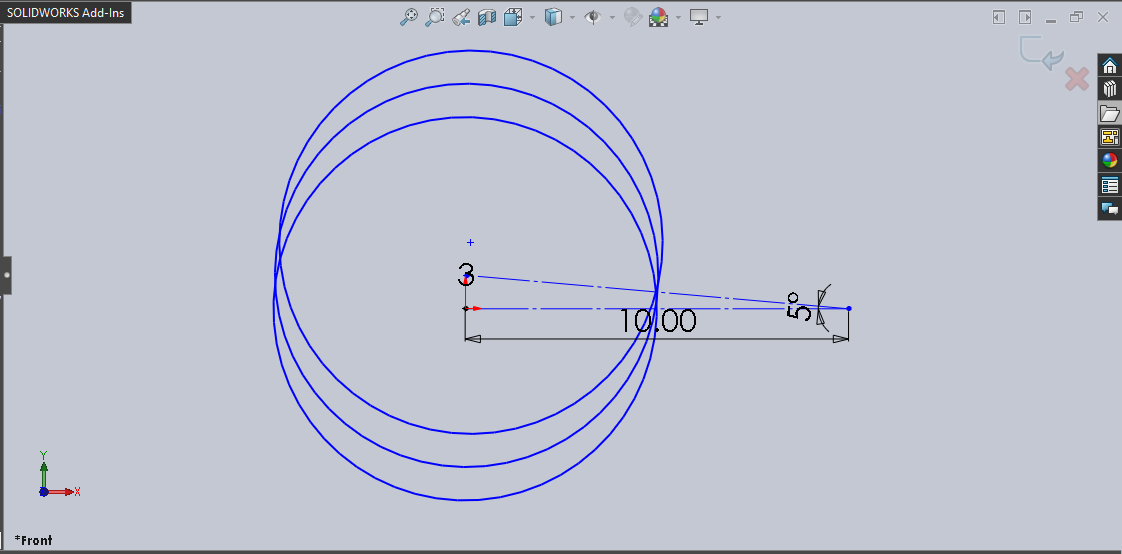``````' De-select the Sketch Segment after Circular Sketch Pattern
swDoc.ClearSelection2 True
``````

In above line we de-select the Sketch Segment after creating Circular Sketch Pattern.

``````' Update Arc Radius
``````

In above line we Update Arc Radius to new value which we will use in Editing previously created Circular Sketch pattern.

Variable Name: `arcRadius`

Updated Value: 20 inch

``````' Edit a Circular Sketch Pattern
BoolStatus = swSketchManager.EditCircularSketchStepAndRepeat(arcRadius, arcAngle, numberOfInstance, patternSpacing, True, "", True, True, True, "Arc1_")
``````

For “editing” a Circular Sketch pattern, we need `EditCircularSketchStepAndRepeat` method from Solidworks Sketch Manager object/variable.

This `CreateCircularSketchStepAndRepeat` method takes following parameters as explained:

• ArcAngle : Angle relative to the sketch entities being patterned. This value is in radian.

• PatternNum : Total number of instances, including the seed geometry.

• PatternSpacing : Spacing between pattern instances. This value is in radian.

• PatternRotate : True to rotate the pattern, false to not.

• DeleteInstances : Number of instances to delete, passed as a string in the format: “(a) (b) (c)”.

• RadiusDim : True to display the radius dimension in the graphics area, false to not.

• AngleDim : True to display the angle dimension between axes in the graphics area, false to not.

• CreateNumOfInstancesDim : True to display the number of instances dimension in the graphics area, false to not.

• Seed: List of the names of the entities, separated by the underscore character (_), that comprise the seed pattern (e.g., Arc1_ as a seed pattern).

NOTE: In Seed, adding underscore(_) after selected entity is important, otherwise code will note work.

After the function complete following are the results:

Return Value:

• True: If Edit Circular Sketch Pattern is *Success.*

• False: If Edit Circular Sketch Pattern is *Fail.*

### Cases

In this section, we will go through different cases by

• Modifying different parameters

• See images, before and after parameter modification

#### CASE 1 : Update Arc Radius

In our code, if we want to update Arc Radius, then we need to update `arcRadius` variable only.

``````' Update Arc Radius
``````

In above line we Update Arc Radius to new value. of 20 inch.

Example Images:

Below image shows before and after we update Arc Radius.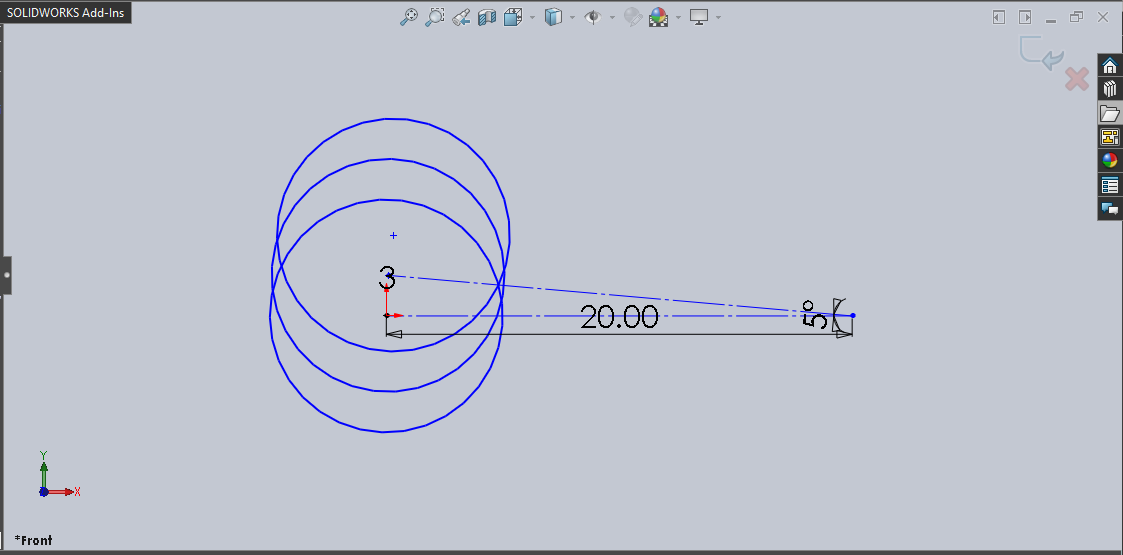#### CASE 2 : Update Arc Angle

In our code, if we want to update Arc Angle, then we need to update `arcAngle` variable only.

``````' Update Arc Angle
arcAngle = 30 * AngleConversionFactor
``````

In above line we Update Arc Angle to new value of 30 inch.

Example Images:

Below image shows before and after we update Arc Angle.

Before Update Arc AngleAfter Update Arc Angle#### CASE 3 : Update Number of Instances

In our code, if we want to update Number of Instances, then we need to update `numberOfInstance` variable only.

``````' Update Number of Instances
numberOfInstance = 5
``````

In above line we Update Number of Instances to new value of 5 number of instances.

Example Images:

Below image shows before and after we update Number of Instances.

Before Update Number of InstancesAfter Update Number of Instances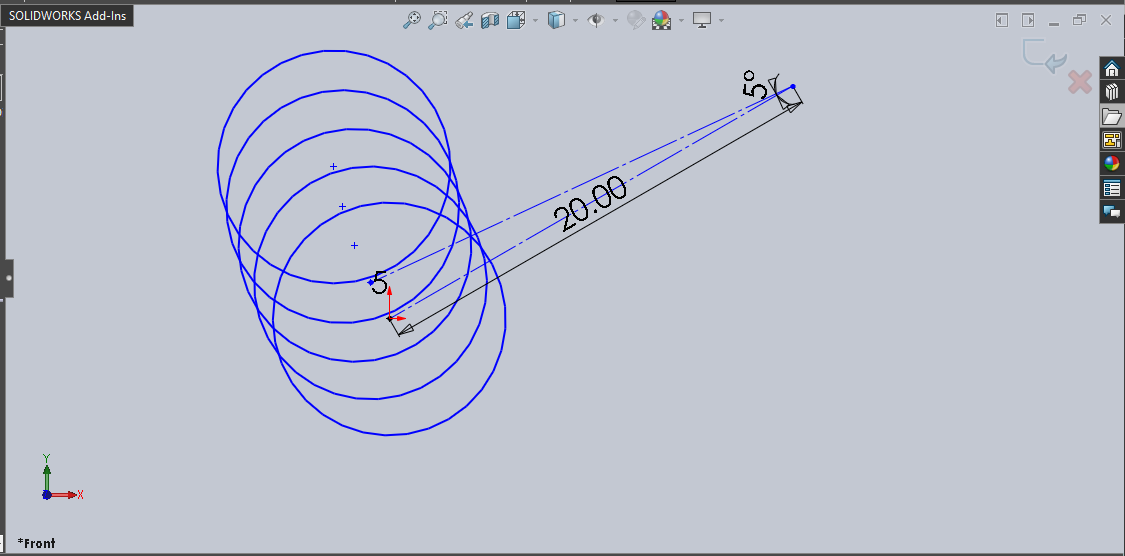#### CASE 4 : Update Pattern Spacing

In our code, if we want to update Number of Instances, then we need to update `patternSpacing` variable only.

``````' Update Pattern Spacing
patternSpacing = 10 * AngleConversionFactor
``````

In above line we Update Pattern Spacing to new value of 10 degree.

Example Images:

Below image shows before and after we update Pattern Spacing.

Before Update Pattern SpacingAfter Update Pattern Spacing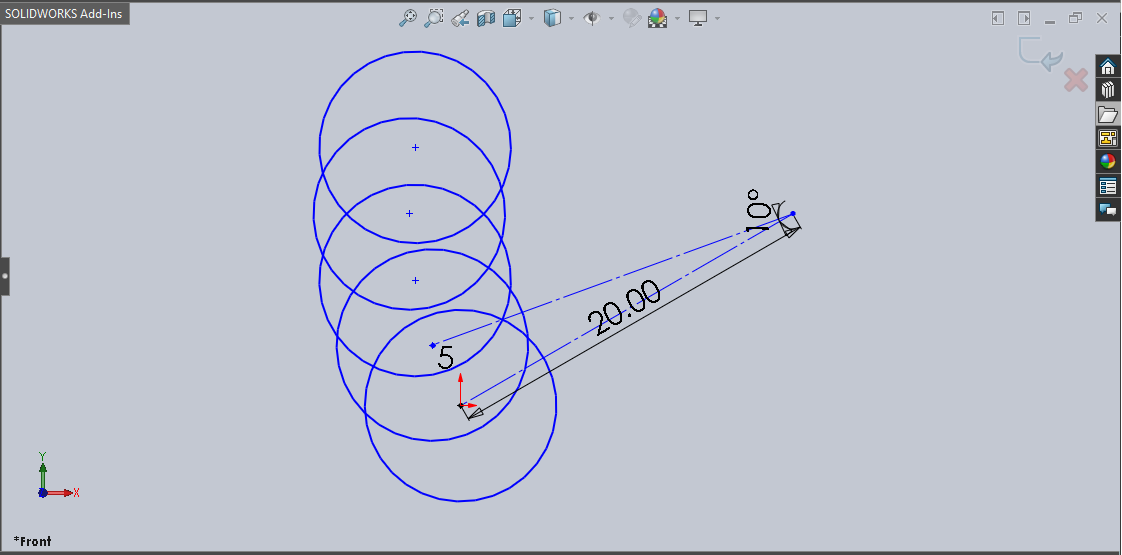#### CASE 5 : Update Display Rotation of Pattern

If we want to update Display Rotation of Pattern, then we need to update value to either `True` or `False`.

In our code, we set this value to `True` which means we are displaying the rotation of pattern.

We update our code for not displaying the rotation of pattern as given in below code sample.

``````' Edit a Circular Sketch Pattern
BoolStatus = swSketchManager.EditCircularSketchStepAndRepeat(arcRadius, arcAngle, numberOfInstance, patternSpacing, False, "", True, True, True, "Arc1_")
``````

Example Images:

Below image shows before and after we update Display Rotation of Pattern.

Before Update Display Rotation of PatternAfter Update Display Rotation of Pattern#### CASE 6 : Update Number of Instances to Delete

If we want to update Number of Instances to Delete, then we need to update value of `""` as given in below code sample.

``````' Edit a Circular Sketch Pattern
BoolStatus = swSketchManager.EditCircularSketchStepAndRepeat(arcRadius, arcAngle, numberOfInstance, patternSpacing, False, "(3)", True, True, True, "Arc1_")
``````

In above code sample, we want to delete 3rd instance hence we pass the number `3` inside `()`.

Note: For delete any instance we need to pass its position in paranthesis (). Otherwise it won’t work.

Example Images:

Below image shows before and after we update Number of Instances to Delete.

Before Update Number of Instances to DeleteAfter Update Number of Instances to Delete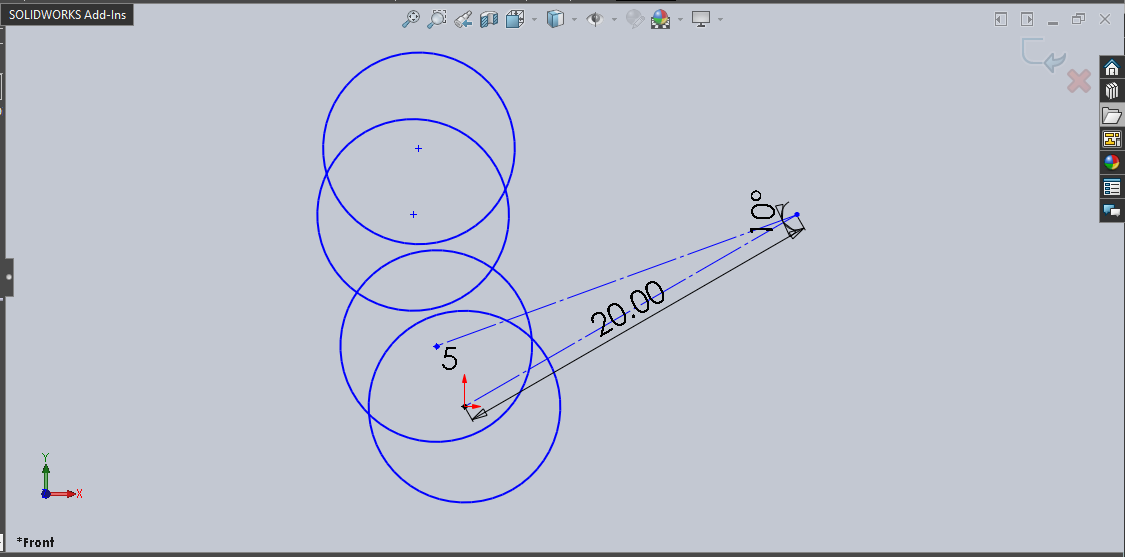#### CASE 7 : Update Display Radius Dimension

If we want to update Display Radius Dimension, then we need to update value to either `True` or `False`.

In our code, we set this value to `True` which means we are displaying the Display Radius Dimension.

We update our code for not displaying the Display Radius Dimension as given in below code sample.

``````' Edit a Circular Sketch Pattern
BoolStatus = swSketchManager.EditCircularSketchStepAndRepeat(arcRadius, arcAngle, numberOfInstance, patternSpacing, False, "(3)", False, True, True, "Arc1_")
``````

Example Images:

Below image shows before and after we update Display Radius Dimension.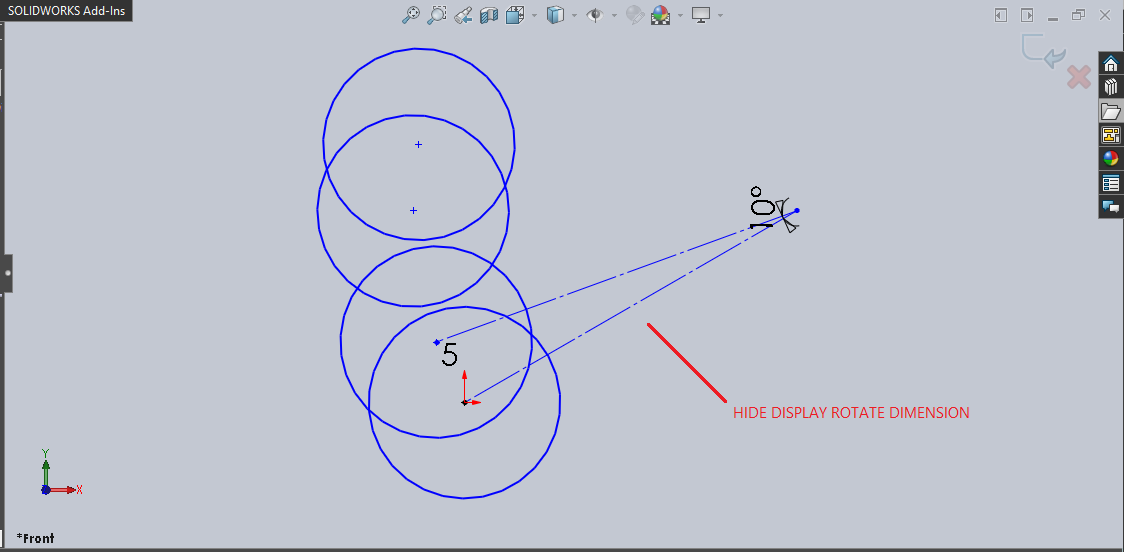#### CASE 8 : Update Display Angle Dimension

If we want to update Display Angle Dimension, then we need to update value to either `True` or `False`.

In our code, we set this value to `True` which means we are displaying the Display Angle Dimension.

We update our code for not displaying the Display Angle Dimension as given in below code sample.

``````' Edit a Circular Sketch Pattern
BoolStatus = swSketchManager.EditCircularSketchStepAndRepeat(arcRadius, arcAngle, numberOfInstance, patternSpacing, False, "(3)", False, False, True, "Arc1_")
``````

Example Images:

Below image shows before and after we update Display Angle Dimension.

Before Update Display Angle DimensionAfter Update Display Angle Dimension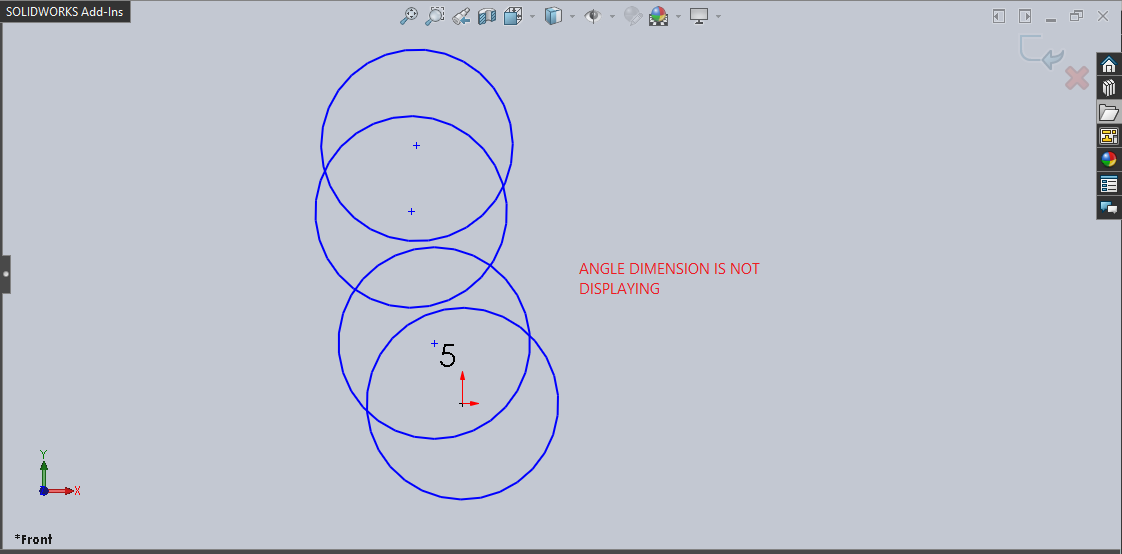#### CASE 9 : Update Display Number of Instances

If we want to update Display Number of Instances, then we need to update value to either `True` or `False`.

In our code, we set this value to `True` which means we are displaying the Display Number of Instances.

We update our code for not displaying the Display Number of Instances as given in below code sample.

``````' Edit a Circular Sketch Pattern
BoolStatus = swSketchManager.EditCircularSketchStepAndRepeat(arcRadius, arcAngle, numberOfInstance, patternSpacing, False, "(3)", False, False, False, "Arc1_")
``````

Example Images:

Below image shows before and after we update Display Number of Instances.

Before Update Display Number of InstancesAfter Update Display Number of Instances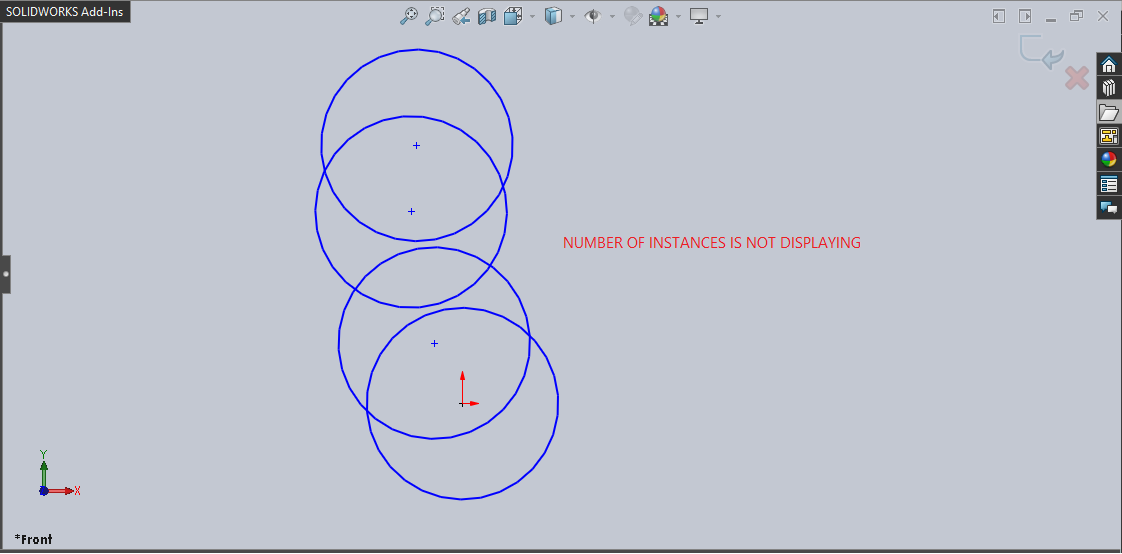This is it !!!

It is indeed a very LONG post. But I try to update the code and move into the direction where we were able to use these code samples in UserForms.

I hope you like my effort!!!

If you found anything to add or update, please let me know on my e-mail.

Hope this post helps you to Edit a Circular Sketch Pattern with Solidworks VBA Macros.

For more such tutorials on Solidworks VBA Macro, do come to this blog after sometime.

If you like the post then please share it with your friends also.

Do let me know by you like this post or not!

Till then, Happy learning!!!

Updated: